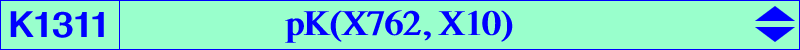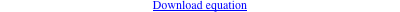too complicated to be written here. Click on the link to download a text file.X(10), X(12), X(37), X(201), X(210), X(594), X(756), X(53008), X(53009), X(53010) Geometric properties :K366 is the locus of pseudo-pivots of central psKs with center X(10). The locus of pseudo-poles is K1311 = pK(X762, X10) and the locus of pseudo-isopivots is K1312 = pK(X1500, X10). One of these cubics is K033 and all four cubics are equivalent to K002. See Table 21. K1311 is the barycentric products X(10) × K345, X(37) × K366, X(321) × K362, X(594) × K317, X(1089) × K319, X(2321) × K964.